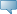# Lebanese American University WWW Information System

## Catalog Entries

Date
Sep 30, 2023Select the Course Number to get further detail on the course. Select the desired Schedule Type to find available classes for the course.

 MTH 102 - Calculus II This course covers integration. Topics include indefinite integrals, integral rules, integration by substitution, estimating with finite sums, Riemann sums and definite integrals, the fundamental theorem of Calculus, substitution in definite integrals, applications of integrals (areas between curves and volumes by slicing, volumes by cylindrical shells, and lengths of plane curves), transcendental functions (logarithms, exponential functions, inverse trigonometric functions), and some basic techniques of integration (integration by parts, and trigonometric integrals). In addition, the course covers differential equations and modeling (first order separable differential equations, linear first order differential equations), vectors in the plane and in space, as well as dot and cross products, lines and planes in space, and conics (ellipse, hyperbola, parabola). 0.000 TO 3.000 Credit hours 3.000 Lecture hours Levels: Undergraduate Schedule Types: Lab, Lecture, Tutorial Computer Science & Mathematics Department Course Attributes: Freshman Science Requirement